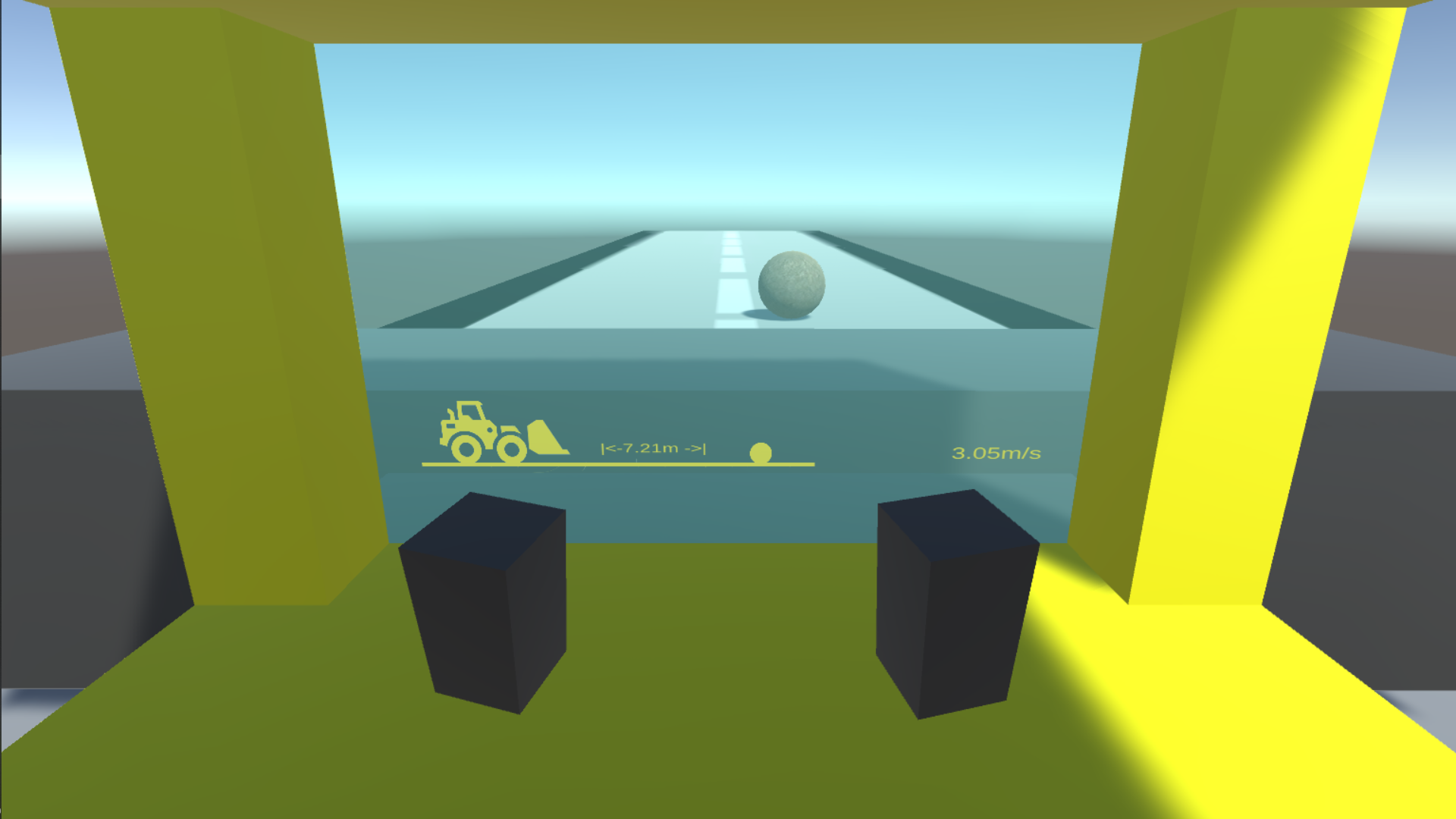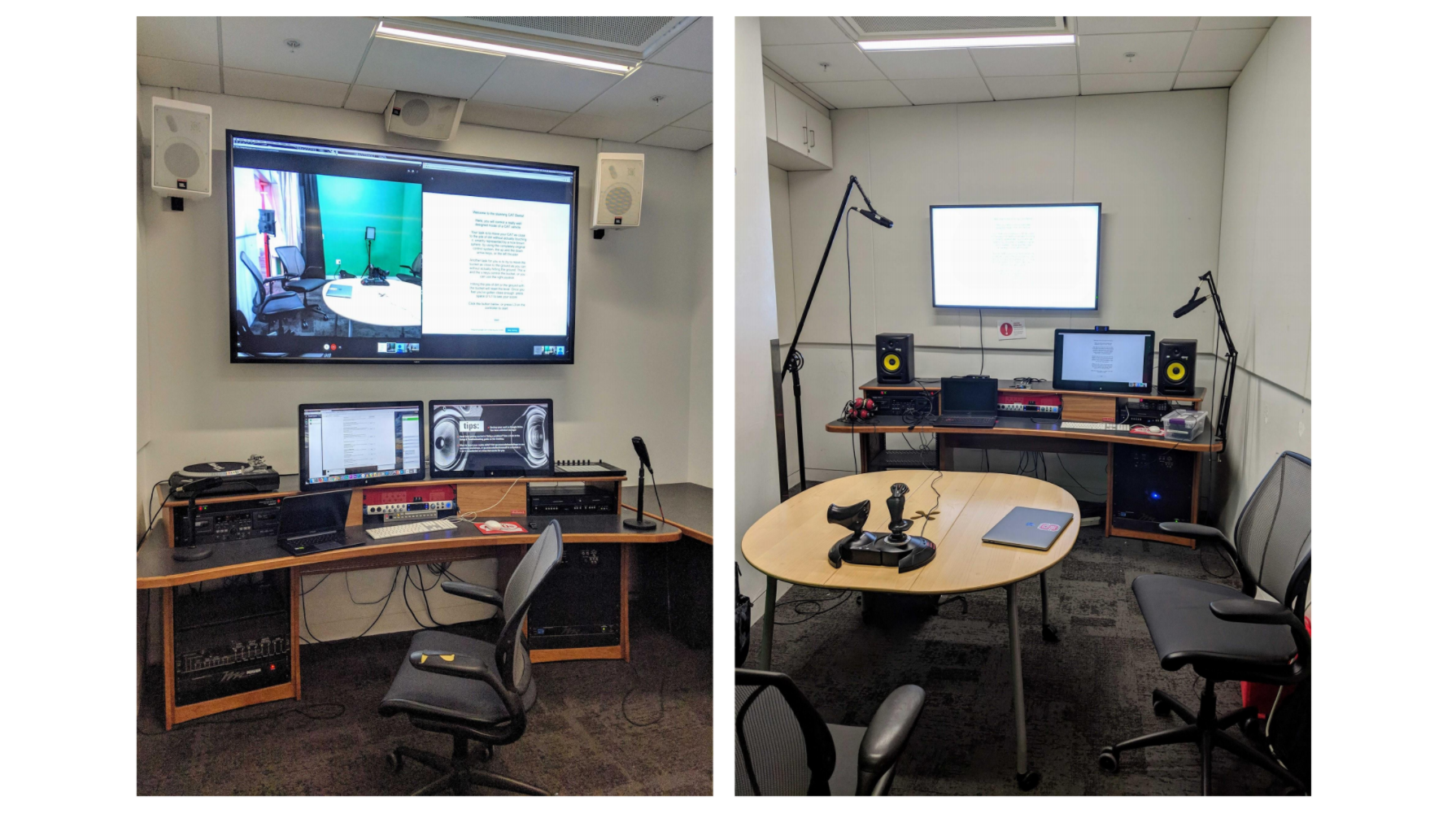## Nonogramz

A puzzle-based role playing game.

``````using System.Collections;
using System.Collections.Generic;
using System.IO;
using UnityEditor;
using UnityEngine;

public class ImageToPuzzle : MonoBehaviour
{

//Source image to create a nonogram of
public GameObject image;

//Width in pixels of the source image, feed only square images for this to work
private int image_size;

//Sprite component of image
private Sprite mySprite;

//2D matrix of size: image_size. Stores colour of each pixel in respective elements
private Color[,] table;

//Size of the puzzle to be created, should be specified in editor
public int puzzle_size;

//2D matrix of puzzle_size, holds colour of each tile of the nonogram
private Color[,] puzzle;

//Size of each block of the puzzle, shows the number of pixels to combine into one block per row/column
private int block_size;

//Prefab of square used to display generated nonogram on screen for testing purposes
public GameObject tile;

// Start is called before the first frame update
void Start()
{

}

//Samples the image pixel by pixel and stores the colour in a separate element in the table matrix
void GenerateImageTable()
{
mySprite = image.GetComponent<SpriteRenderer>().sprite;
image_size = (int)mySprite.rect.width;
table = new Color[image_size, image_size];
for (int i = 0; i < image_size; i++)
{
for (int j = 0; j < image_size; j++)
{
table[i, j] = mySprite.texture.GetPixel(j, image_size - i - 1);
}
}
}

//Cuts down the table matrix into desired puzzle size matrix
void GeneratePuzzleTable()
{
block_size = image_size / puzzle_size;
puzzle = new Color[puzzle_size, puzzle_size];
GameObject tileDisplay = new GameObject("TileDisplay");

for (int i = 0; i < puzzle_size; i++)
{
for (int j = 0; j < puzzle_size; j++)
{
puzzle[i, j] = Avg(i * block_size, j * block_size);
GameObject x = Instantiate(tile, new Vector3(1 + j * (float)0.30, 1 - i * (float)0.30, 0), Quaternion.identity);
x.transform.SetParent(tileDisplay.transform);
x.GetComponent<Renderer>().material.color = puzzle[i, j];
}
}
}

//Creates the puzzle's text file from collected information
void GeneratePuzzleText()
{
int count = 1;
string filepath = Application.dataPath + "/Resources/Nonograms/Generated/" + puzzle_size.ToString() + "x" + puzzle_size.ToString() + image.name;
if (!File.Exists(filepath + ".txt"))
{
using (StreamWriter sw = File.CreateText(filepath + ".txt"))
{
sw.WriteLine(puzzle_size.ToString());
sw.WriteLine(getColNumberText());
sw.WriteLine(puzzle_size.ToString());
sw.WriteLine(getRowNumberText());
sw.WriteLine(MapColors());
sw.WriteLine(image.name);
}
}
else
{
while (File.Exists(filepath + count.ToString() + ".txt"))
{
count++;
}
using (StreamWriter sw = File.CreateText(filepath + count.ToString() + ".txt"))
{
sw.WriteLine(puzzle_size.ToString());
sw.WriteLine(getColNumberText());
sw.WriteLine(puzzle_size.ToString());
sw.WriteLine(getRowNumberText());
sw.WriteLine(MapColors());
sw.WriteLine(image.name);
}
}
}

//Generates Column Labels
string getColNumberText()
{
string newString = "";
for (int i = 0; i < puzzle_size; i++)
{
int count = 0;
int commaCount = 0;
if (i > 0)
{
newString += "|";
}
for (int j = 0; j < puzzle_size; j++)
{
if (puzzle[j, i].a == 1)
{
count++;
}
else if (count > 0)
{
if (commaCount > 0)
{
newString += "," + count;
}
else
{
newString += count;
commaCount++;
}
count = 0;
}
}
if (count > 0)
{
if (commaCount > 0)
{
newString += "," + count;
}
else
{
newString += count;
commaCount++;
}
count = 0;
}
else
{
if (commaCount == 0)
{
newString += "0";
}
}
}
return newString;
}

//Generates row labels
string getRowNumberText()
{
string newString = "";
for (int i = 0; i < puzzle_size; i++)
{
int count = 0;
int commaCount = 0;
if (i > 0)
{
newString += "|";
}
for (int j = 0; j < puzzle_size; j++)
{
if (puzzle[i, j].a == 1)
{
count++;
}
else if (count > 0)
{
if (commaCount > 0)
{
newString += "," + count;
}
else
{
newString += count;
commaCount++;
}
count = 0;
}
}
if (count > 0)
{
if (commaCount > 0)
{
newString += "," + count;
}
else
{
newString += count;
commaCount++;
}
count = 0;
}
else
{
if (commaCount == 0)
{
newString += "0";
}
}
}
//Debug.Log(newString);
return newString;
}

//Creates a cut-down version of the Puzzle matrix according to the txt format
string MapColors()
{
List<string> colors = new List<string>();
int[,] newPuzzle = new int[puzzle_size, puzzle_size];
string matrix_ = "";

for (int i = 0; i < puzzle_size; i++)
{
for (int j = 0; j < puzzle_size; j++)
{
if (puzzle[i, j].a > 0)
{
if (!colors.Contains(ColorUtility.ToHtmlStringRGB(puzzle[i, j])))
{
}
newPuzzle[i, j] = colors.IndexOf(ColorUtility.ToHtmlStringRGB(puzzle[i, j])) + 1;
}
else
{
newPuzzle[i, j] = 0;
}
matrix_ += newPuzzle[i, j].ToString() + " ";
}
matrix_ += "\n";
}

string cols = colors.Count.ToString();
foreach (string clr in colors)
{
cols += " " + clr;
}
matrix_ = cols + "\n" + matrix_;
return matrix_;

}

//Returns the average colour value from a block_size x block_size group in the image table
Color Avg(int row, int col)
{
Color sum = new Color();
for (int i = row; i < row + block_size; i++)
{
for (int j = col; j < col + block_size; j++)
{
sum += table[i, j];
}
}
return sum / (block_size * block_size);
}

// Update is called once per frame
void Update()
{
GenerateImageTable();
GeneratePuzzleTable();
GeneratePuzzleText();
Destroy(this); //The generation has to be done only once, so destroy this script after one loop
}
}```
```

Nonogramz is an RPG where a player progresses through the story by solving nonogram puzzles. It is being developed on Unity3D for mobile and PC platforms. I primarily worked on the automation of the creator's engine, where the gamemakers can upload any image and the game converts it into a puzzle, which can later be used during actual gameplay. The code sample above shows how I've dealt with reading colors, pixels and more.

Nonogramz is the first Unity3D game that I worked on that was designed to be played (all others were for research/simulation purposes). I worked with a team of around 15 people, and closely followed the Agile methodolody through this project. I experienced how different teams interact, how the workflow progresses between the design team and the development team. I was leading new team members within days of joining the project thanks to my active contribution and ability to communicate well, and I am still one of two admins overseeing the development of this game.

## M.A.P.S - NPC Behaviour

A game designed for observing how different kinds of NPCs interact with each other.

``````using Pada1.BBCore.Tasks;
using UnityEngine;

namespace BBUnity.Actions
{
/// <summary>
/// It is an action to find the closest game object with a given label.
/// </summary>
[Action("MyActions/IsTargetCloseSmart")]
[Help("Returns true if target is closer than given distance.")]
public class IsTargetCloseSmart : GOAction
{
///<value>Input Target Parameter to to check the distance.</value>
[InParam("target")]
[Help("Target to check the distance")]
public GameObject target;

///<value>Input maximun distance Parameter to consider that the target is close.</value>
[InParam("closeDistance")]
[Help("The maximun distance to consider that the target is close")]
public float closeDistance;

private bool completed;
/// <summary>Initialization Method of ClosestGameObjectWithTag.</summary>
/// <remarks>Get all the GamesObject with that tag and check which is the closest one.</remarks>
public override void OnStart()
{
if (target == null)
{
completed = false;
}
else
{
if ((gameObject.transform.position - target.transform.position).sqrMagnitude < closeDistance * closeDistance)
{
completed = true;
}
else
{
completed = false;
}
}
}
/// <summary>Method of Update of ClosestGameObjectWithTag.</summary>
{
if (completed)
{
//Debug.Log("Passed");
}
else
{
//Debug.Log("Failed");
}
}
}
}
```
```

To learn more about the intelligence that goes into games, I built M.A.P.S (Meek.Aggressive.Player.Smart) with two fellow students at NC State. M.A.P.S is a game made in Unity3D meant to simulate the interactions between different kinds of behaviours assigned to different factions in a simple attack-and-conquer style game. Deciding the way each unit 'thinks' and putting it into practice helped me become thorough in my logical reasoning. Observing the unintended emergent behaviours of the units was fascinating as I saw entirely different playstyles arise from slight changes in the decision making process of the NPCs.

The video above shows how the Meek (blue), Aggressive (green) and Smart (cyan) units interact with each other, which is a really interesting watch. A behaviour tree representation of one of those factions - Smart - is shown above as well. The code sample shows how one of those numerous actions is implemented. Having control over how each and every unit reacts was a very compelling experience, and was one of the key factors in making me interested in video games.

## Transparent Buckets

The complete lifecycle of an interactive User Experience demonstrationThis project was a great learning experience for me as we ran through an entire software development life cycle using the Sprint Methodology. Going through each phase working with a team consisting of various perspectives and ideas was super exciting. While this was just academic project that required students to discuss about a problem and propose a solution to it, my familiarity in Unity helped my team come up with a working prototype that closely simulated the concept we were assigned.

This project gave me a wholistic exerience on working with people who each specialized in wildly different skillsets, and being able to bring them all together for this final product helped me get a feel of leading teams as well as being a part of one. This project simulates the first-person view of a construction vehicle's operator, and the problems faced by them. It was programmed to use a flight controller, which happened to be the closest equipment we could get to the actual vehicles used in the field. Working on a project that placed entirety of its focus on the user taught me plenty on how to understand what the end-user wants.